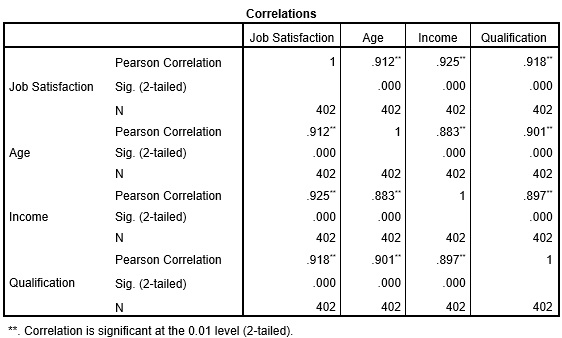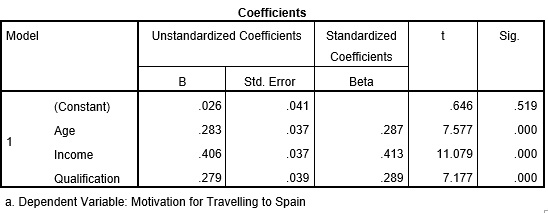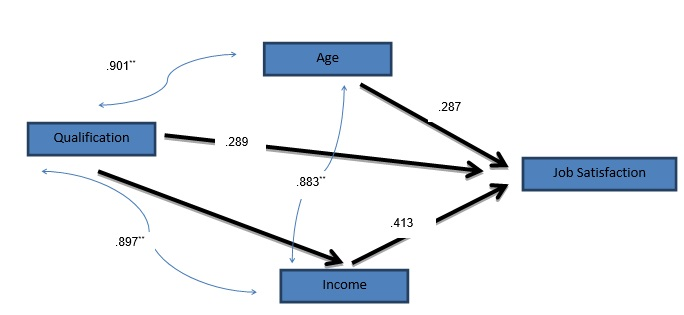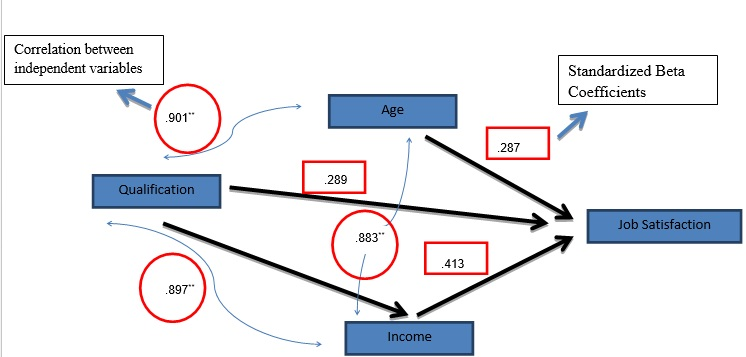# How to conduct path analysis?

Path analysis is a graphical representation of multiple regression models. In this analysis, the graphs represent the relationship between the dependent and independent variables with the help of square and arrows. These graphs show the causation between different variables of a regression model. To conduct a path analysis, simply write the names of variables in square boxes and connect the square boxes with arrows. This will indicate the effect of one on another, similar to regression. Path analysis takes effect in two ways; before and after running the regression. They are also known as ‘input path diagram’ and ‘output path diagram’ respectively. While the input path diagram represents the causal connections between variables while proposing a hypothesis, the output path diagram shows the actual outcome of the test. These outcomes help test the hypothesis propose in input path diagrams.

## Understanding path analysis

In this case study, there are three independent variables; ‘age’, ‘income’ and ‘qualification’. These variables affect the ‘job satisfaction’ of employees. Therefore, ‘job satisfaction’ becomes the dependent variable and the others become the independent variables. The hypotheses for this case are:

• Hypothesis 1: Qualification has a positive impact on job satisfaction.
• Hypothesis 2: Income has a positive impact on job satisfaction.
• Hypothesis 3: Age has a negative impact on job satisfaction.

Firstly, construct the input path diagram to show the causal connections put forth in these hypotheses. The path diagram for the hypotheses appears as under:Figure 1: a Hypothesized input path analysis

The above input diagram displays an intuitive idea of how age, qualification and income affect job satisfaction. Since one of the above hypotheses proposes a negative impact of age on job satisfaction, that line is red. The diagram is simple and assumes all three variables have a direct impact on the dependent variable.

In the above case, input path analysis shows the hypothesized relationship. Furthermore, it only includes the effect of the independent variable on the dependent variables. However, these three independent variables can also have an effect on each other. Therefore, output path diagrams can be constructed after performing correlation and regression analysis. The items undertaken by output path analysis include:

## Correlation coefficients

SPSS software is used for analysing this case. The images below show the correlation between the variables in the model. ‘Sig. (2-tailed)’ indicates the significance of the correlation coefficient. The correlation coefficients are significant if the significance value is less than or equal to 0.05.Results from Pearson correlation analysis conducted in SPSS

## Standardized beta coefficients in regression results

A standardized beta coefficient is important for path diagrams because it represents a direct impact of an independent variable on the dependent variable. The regression results have been shown in below two images.Regression results from SPSSRegression results from SPSS

Here, the regression results components taken for output path analysis includes standardized beta coefficients and correlation coefficients. The output path analysis will appear as under:Figure 2: Output path analysis based on correlation coefficients

Furthermore, the below given numbers represent the correlation between independent variables and standardized beta coefficients.Figure 3: Output path analysis based on correlation and regression coefficients

On the basis of output path analysis, the conclusion is that the prediction about the positive and significant impact of income and qualification is true. However, the negative impact of age on job satisfaction is rejected as the regression coefficient of age is positive.

## Application of path analysis

Since path analysis is the extension of regression analysis, it is only useful in cases applying regression analysis techniques.

## Software supporting path analysis

Software that supports this analysis with multiple regressions is R, SAS, MATLAB, STATA, and SPSS (AMOS version only).

### Indra Giri

Senior Analyst at Project Guru
He completed his Masters in Development Economics from South Asian University, New Delhi. His areas of interest includes various socio development issues like poverty, inequality and unemployment in South Asia. Apart from writing for Project Guru he loves to travel and play football in his spare time.

### Related articles

• Chi square test with the help of SPSS A chi square (X2) statistic is used to investigate whether distributions of categorical variables differ from one another. Categorical variables like; gender of sample population which could be either male or female.
• How to conduct generalized least squares test? In statistics, Generalized Least Squares (GLS) is one of the most popular methods for estimating unknown coefficients of a linear regression model when the independent variable is correlating with the residuals.
• How to apply logistic regression in a case? Machine learning involves solutions to predict scenarios based on past data. Logistic regression offers probability functions based on inputs and their corresponding output.
• How to perform nonlinear regression? Regression analysis is a statistical tool to study the relationship between variables. These variables are the outcome variable and one or more exposure variables. In other words, regression analysis is an equation which predicts a response from the value of a certain predictor.
• How to perform LASSO regression test? In statistics, to increase the prediction accuracy and interpret-ability of the model, LASSO (Least Absolute Shrinkage and Selection Operator) is extremely popular. It is a regression procedure that involves selection and regularisation and was developed in 1989. Lasso regression is an […]
Discussions

1.Joseph M. Bika

Giri, i appreciate your explanation on path analysis. But how can i get the SPSS Amos version? thanks

•priya

SPSS Amos is a paid software and can be purchased from their website

2.Htet Htet Oo

Path analysisWe are looking for candidates who have completed their master's degree or Ph.D. Click here to know more about our vacancies.